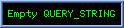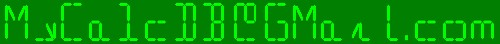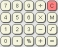A Big database for small calculators.

Last database update :
04-21-2014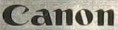Canon  FS-400  aka SCIENTIFIC STATISTICAL CALCULATOR   (4 pictures)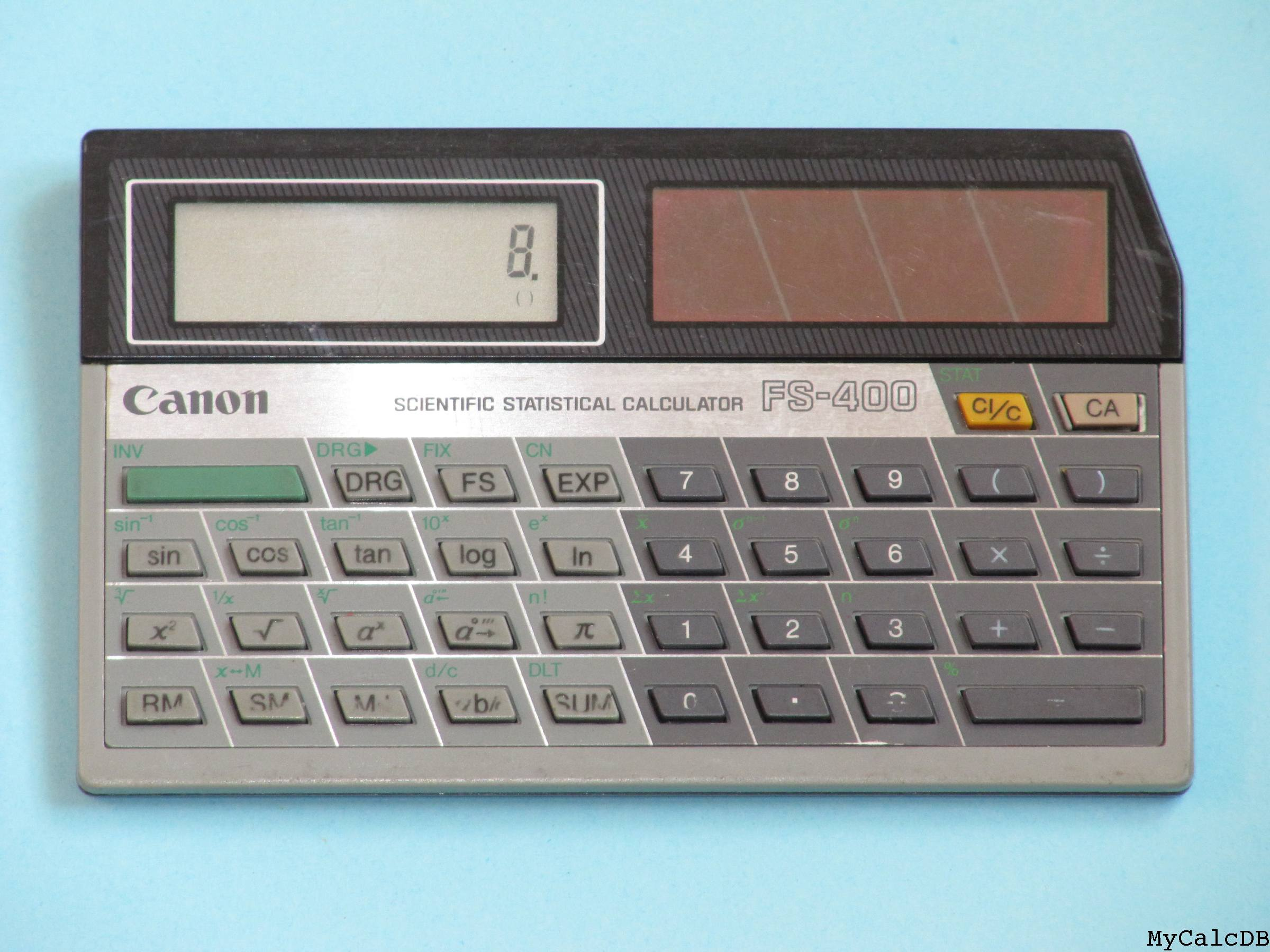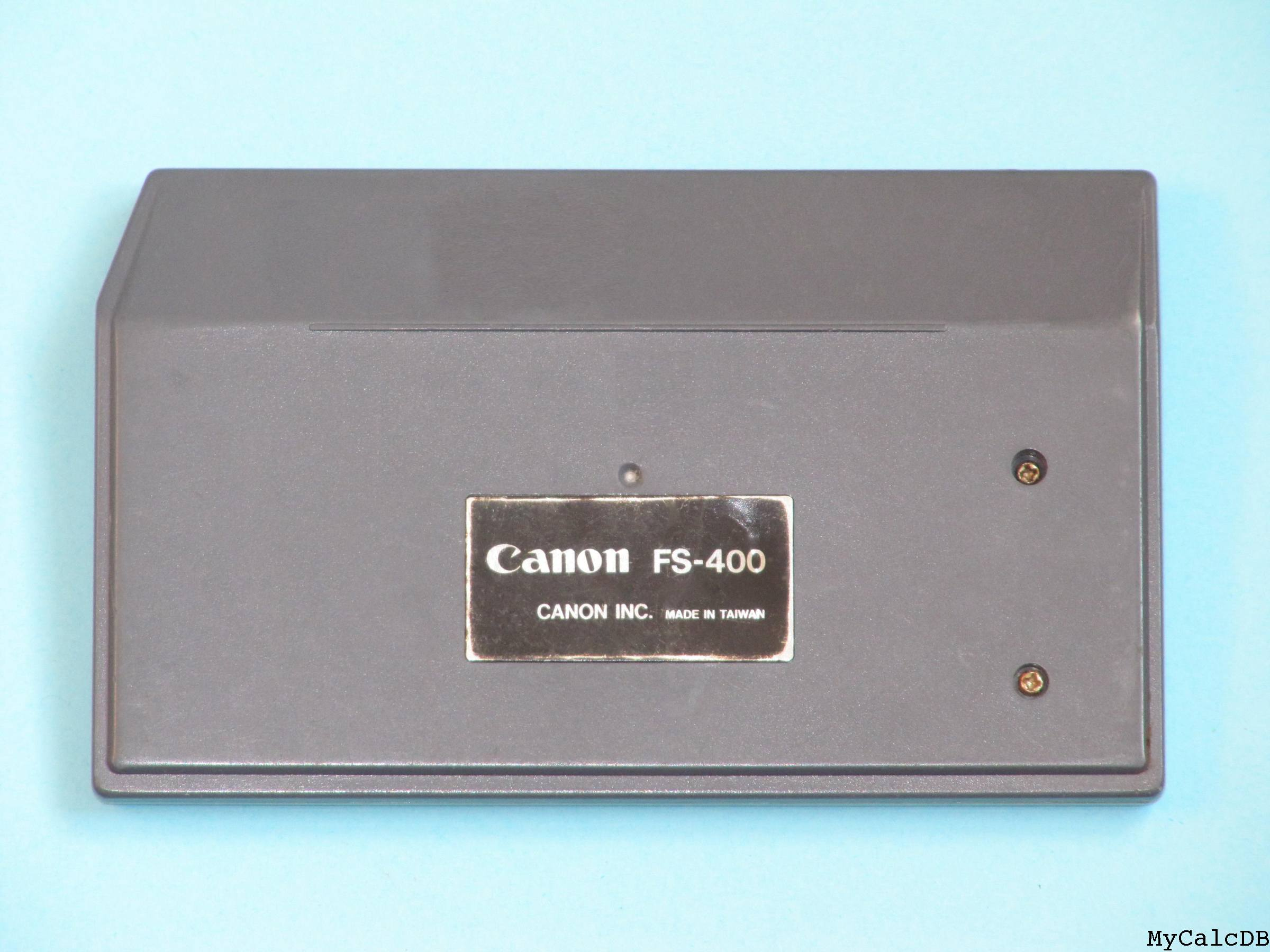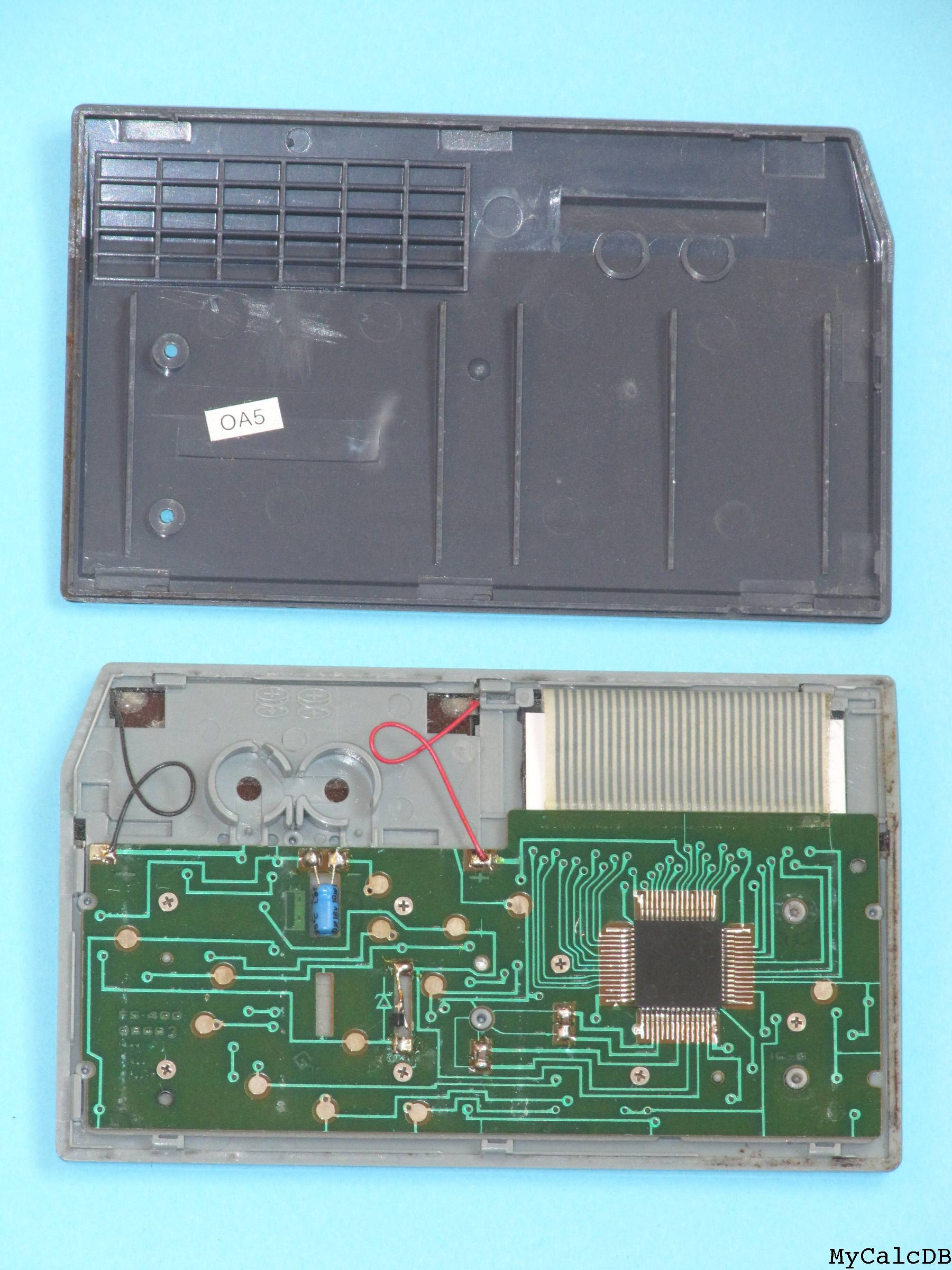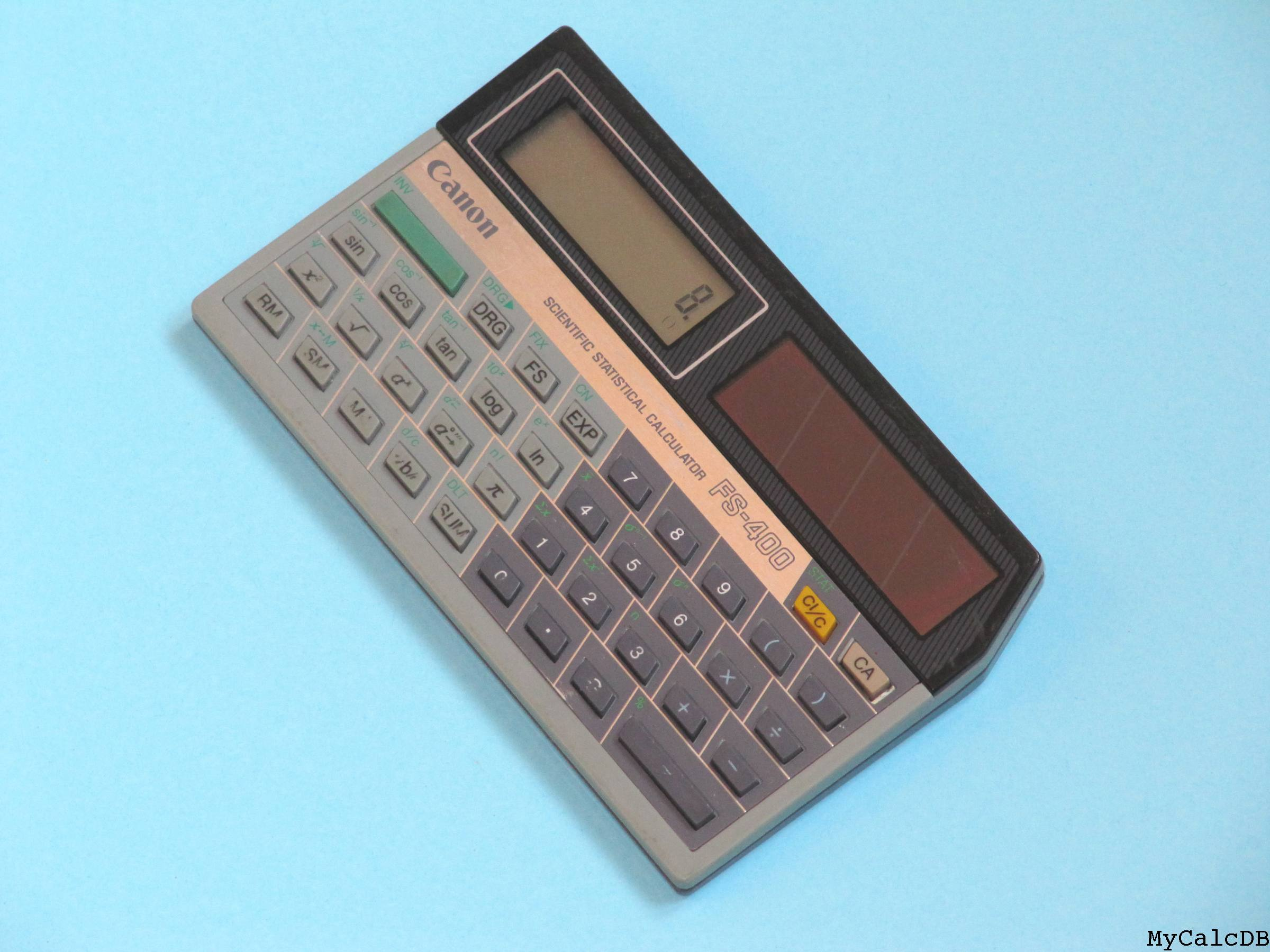Introduction date~1986
End date
TypeScient+Stat
Last update date2010_01_02
ProgrammableN
RAM size1
ROM size
Number of functions36+4mem+8stat
Alpha capabilitiesN
Number of keys40
Display typeLCD
Number of digits on display8 or 6+2 + indic
Graphical (X x Y)N
Extention(s)N
PrinterN
SavingN
Date or clock capabilitiesN
Microprocessor
Functions list"DRG FS EXP ( ) sin cos tan log ln x^2 Sqrt a^x a°'""-> Pi a_b/c +/- DRG-> FIX CN asin acos atan 10^x e^x x^(1/3) 1/x x^(1/y) a°'""<- n! d/c % RM SM M+ SUM STAT meanx sigma^n-1 sigma^n Sumx Sumx^2 n DLT"
Size (length x width x thickness)81x134x16
Weight
BatteriesN
External powersolar
Power consumption
Twin